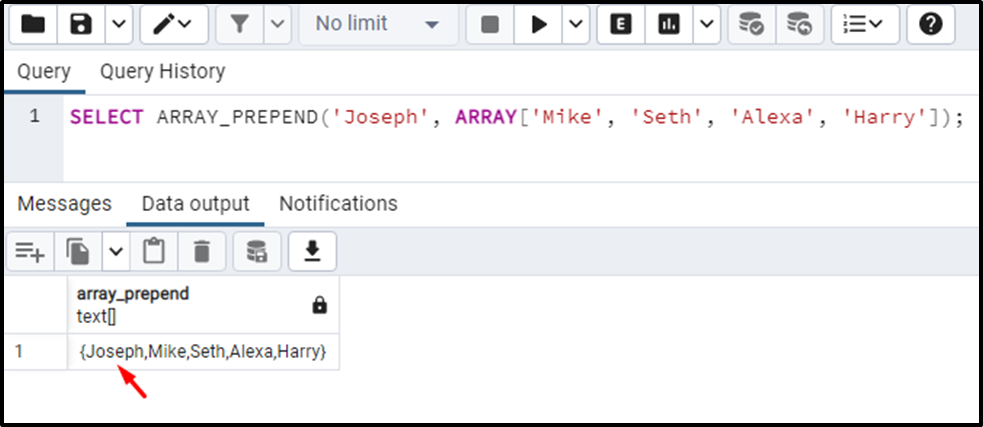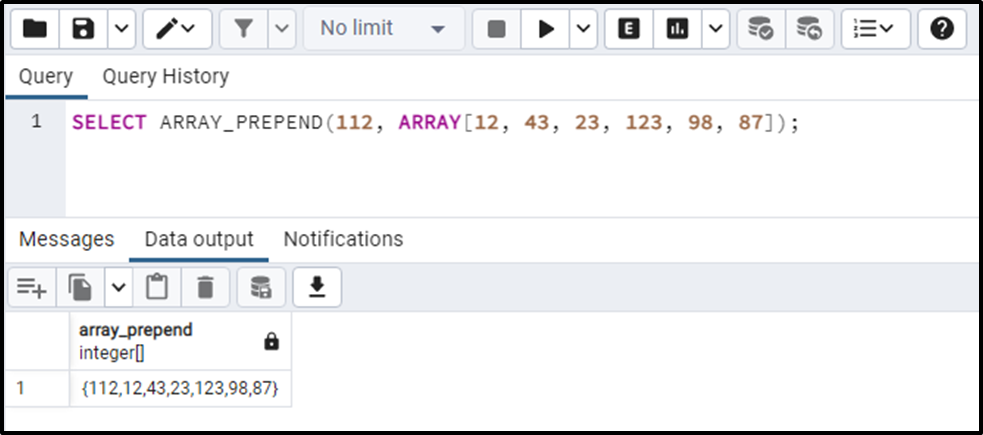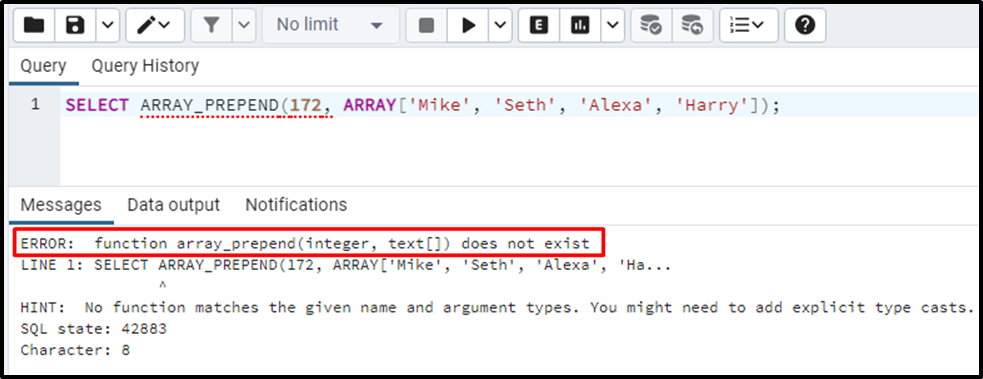##### PostgreSQL ARRAY_PREPEND() Function

PostgreSQL provides several built-in functions that allow us to work with the arrays. For instance, the ARRAY_LENGTH() retrieves the length of an array along with a particular dimension, the ARRAY_CAT() function concatenates two arrays, the ARRAY_APPEND() function appends an element to the end of an array, etc. The ARRAY_PREPEND() is one of the array functions that append an element at the start of an array.

Using suitable examples, this blog post will teach you how to use the ARRAY_PREPEND() function in Postgres. So, let’s start!

How to Use ARRAY_PREPEND() Function in Postgres?

Postgres users can use the ARRAY_PREPEND() function to append an element at an array's starting index. To avail the functionality of the ARRAY_PREPEND() function, users must use the following syntax:

`ARRAY_PREPEND(item, arr);`

"item" represents any element to be appended to an array at its starting index. While "arr" represents the array to which the element will be appended.

Example 1: How to Prepend a String Type Element to an Array in Postgres?

Suppose we want to append the name “Joseph” at the start of an array; to do this, we will use the ARRAY_PREPEND() function as follows:

`SELECT ARRAY_PREPEND('Joseph', ARRAY['Mike', 'Seth', 'Alexa', 'Harry']);`The output snippet shows that the given element has been appended to the array at the beginning.

Example 2: How to Prepend an INTEGER Element to an Array in Postgres?

If you want to append any integer to the start of an array, then you can use the ARRAY_PREPEND() function as follows:

`SELECT ARRAY_PREPEND(112, ARRAY[12, 43, 23, 123, 98, 87]);`The output shows that an integer value “112” has been appended at the start of the input array.

Example 3: Prepend an INTEGER With String Array

If a user tries to prepend a string value with an integer array or vice versa, then Postgres will throw a “function does not exist” error.

`SELECT ARRAY_PREPEND(172, ARRAY['Mike', 'Seth', 'Alexa', 'Harry']);`The output snippet proves that arbitrary data types cannot be prepended to an array of a particular type.

That’s all! From this Postgres blog!

Conclusion

PostgreSQL provides a built-in ARRAY_PREPEND() function that is used to append an element at the start of an array. It accepts two arguments: an element and an array; consequently, the ARRAY_PREPEND() function prepends the given element at the start of the targeted array. However, if a user tries to prepend a string value with an integer array or vice versa, then Postgres will throw a “function does not exist” error. This post demonstrated the working of the ARRAY_PREPEND() function with various suitable examples.